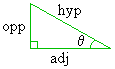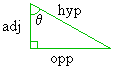Home    |    Teacher    |    Parents    |    Glossary    |    About UsEmail this page to a friendResources· Cool Tools · Formulas & Tables · References · Test Preparation · Study Tips · Wonders of MathSearchFunctions ( Math | Algebra | Functions )

Trig Functions: The FunctionsThe functions are usually abbreviated: sine (sin), cosine (cos), tangent (tan) cosecant (csc), secant (sec), and cotangent (cot).

It is often simpler to memorize the the trig functions in terms of only sine and cosine:

 sine(q) = opp/hyp csc(q) = 1/sin(q) cos(q) = adj/hyp sec(q) = 1/COs(q) tan(q) = sin(q)/cos(q) cot(q) = 1/tan(q)

The functions are usually abbreviated:

arcsine (arcsin)
arccosine (arccos)
arctangent (arctan)
arccosecant (arccsc)
arcsecant (arcsec)
arccotangent (arccot).

According to the standard notation for inverse functions (f-1), you will also often see these written as sin-1, cos-1, tan-1 arccsc-1, arcsec-1, and arccot-1. Beware, though, there is another common notation that writes the square of the trig functions, such as (sin(x))2 as sin2(x). This can be confusing, for you then might then be lead to think that sin-1(x) = (sin(x))-1, which is not true. The negative one superscript here is a special notation that denotes inverse functions (not multiplicative inverses).